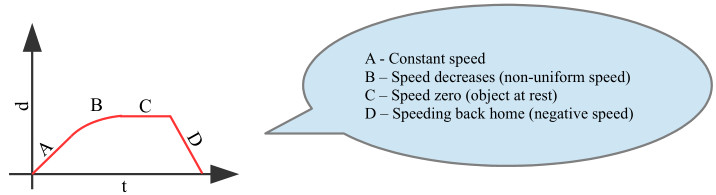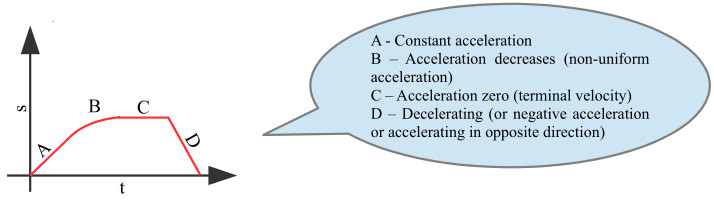Home » Handbook of O Level Physics » UNIT 2: SPEED, VELOCITY AND ACCELERATION

# UNIT 2: SPEED, VELOCITY AND ACCELERATION

• Speed: It is the rate of change of distance with respect to time.

s = d / t

• Velocity: It is the rate of change of displacement with respect to time.

• Acceleration: It is the rate of change of velocity with respect to time.

a = (v – u) / t

• Non-uniform deceleration: It is when there are unequal decreases in velocity in equal intervals of time.

• Graphs can be used to study motion.

• d-t graph

• Speed is equal to the gradient of such a graph.

s = (d2 – d1) / (t2 – t1)

• Example:• s-t graph

• Acceleration is equal to the gradient of such a graph.

a = (s2 – s1) / (t2 – t1)

• Distance covered is equal to the the area under the graph.

• Example:• Constant gradient or uniform speed or uniform acceleration is represented by a straight line.

• Acceleration can happen even when speed is constant if direction is changing.

• For an object going in a circle at constant speed, the resultant force is towards the center of the circle.

• Free-fall

• Without air

• Constant acceleration (of 10 m/s2 on Earth).

• Objects of different weights fall together.

• With air

• As the air resistance increases, the resultant force (of weight and air-resistance) decreases.

• As resultant force decreases, the acceleration also decreases (according to Newton’s Second Law of Motion).

• When the resultant force becomes zero, there is no acceleration and the body is said to be falling with terminal velocity.